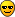# Fitting a Sum Of Gaussians a la Sick(1974)

I’m writing my function as a sum of four gaussians multiplied by the domain so that the function goes to zero AT zero. It does well fitting, but I’m having a hard time doing a proper constraint of the slope at zero and keeping it below a certain value as the domain goes beyond a region for which there is no data. I’m loading a separate file with the function I’m fitting, then I’m calling a TF1 and trying to fit it to a TGraphErrors. The code:

Double_t fm2GeV = 0.197;

Double_t Gaussians(Double_t *Q2, Double_t *par) {

Float_t rnn = par; // slope at zero
Double_t sigmab = 40.0289; // (2sigma)^{2}
Double_t a1 = par;
Double_t a2 = par;
Double_t a3 = par;
Double_t a4 = par;

Double_t q1 = par;
Double_t q2 = par;
Double_t q3 = par;
Double_t q4 = par;

Double_t Gauss1 = a1TMath::Exp(-1.0(sqrt(Q2)-q1)**2/sigmab);
Double_t Gauss2 = a2TMath::Exp(-1.0(sqrt(Q2)-q2)**2/sigmab);
Double_t Gauss3 = a3TMath::Exp(-1.0(sqrt(Q2)-q3)**2/sigmab);
Double_t Gauss4 = a4TMath::Exp(-1.0(sqrt(Q2)-q4)**2/sigmab);

Double_t Gauss10 = a1TMath::Exp(-1.0q12/sigmab); // functions for the constraint of the slope…
Double_t Gauss20 = a2TMath::Exp(-1.0q2
2/sigmab);
Double_t Gauss30 = a3TMath::Exp(-1.0q32/sigmab);
Double_t Gauss40 = a4TMath::Exp(-1.0q4
2/sigmab);

rnn = -6fm2GeV**2(Gauss10+Gauss20+Gauss30+Gauss40); //slope at zero

return Q2*(Gauss1+Gauss2+Gauss3+Gauss4);

}

//In my other Program, this is how I call the TF1…

TF1 *SOG4 = new TF1(“SOG4”,Gaussians,0,1.8,9);
//SOG4->SetLineStyle(1);
SOG4->SetLineWidth(2);
SOG4->SetLineColor(4);
SOG4->SetParameter(0,0.3371);
SOG4->SetParLimits(0,0.3,0.35);
SOG4->SetParameter(1,0.2505);
SOG4->SetParLimits(1,0.24,0.27);
SOG4->SetParameter(2,0.04979);
SOG4->SetParLimits(2,0.045,0.052);
SOG4->SetParameter(3,0.7915);
SOG4->SetParLimits(3,0.75,0.82);
SOG4->SetParameter(4,0.02364);
SOG4->SetParLimits(4,0.02,0.026);
SOG4->SetParameter(5,1.3);
SOG4->SetParLimits(5,1.1,1.4);
SOG4->SetParameter(6,0.05);
SOG4->SetParLimits(7,0.001,0.05);
SOG4->SetParameter(7,0.2);
SOG4->SetParLimits(8,0.0,0.5);
Double_t RN = -0.115;

//And here I Fit to a Graph with the world’s data on GEn and attempt to pull out the covariance matrix.

grWorld->Fit(SOG4,“E”,"",0,1.;
SOG4->SetParError(8,0.005);
gMinuit->mnemat(&SOGmatrix,9);

/// This sum of gaussians NEEDS to match the slope I’m setting. I’m not sure why it’s not matching the constraint with the way I’ve written it… I’d also like to make sure the function stays less than the dipole form that becomes dominant at high Q2 as defined by Friedrich and Walcher … but I don’t know how to establish a “less than” constraint to the fit at high Q2 without putting more fiducial points on the graph.

I figured out how to do what I needed… I already had it correctly written… And I’m just going to use a fiducial point with a large error bar.

I must admit that the original title drew my attention .
A few observations :

1. Your code does neither define or apply a constrain to the slope .
2. How to apply an upper limit to the high Q^2 behavior of the
form factor has been described in detail by Jorg Friedrich in his
articles around 1975-1980 .
3. Any reason for not using the existing codes to perform fitting
of charge distributions .

Last but not least , did you actually expect somebody who was not
familiar to electron scattering lingo to understand your email ??

Eddy